# Excel Applications for Accounting Principles

## Quiz 20 : Activity-Based Costing ABCLooking for Accounting Homework Help?

## Quiz 20 :Activity-Based Costing ABC

Question TypePROBLEM DATA Bumblebee Mobiles manufactures a line of cell phones. The management has identified the following overhead costs and related cost drivers for the coming year.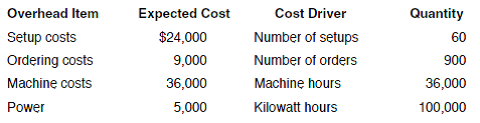The following were incurred in manufacturing two of their cell phones, Bubble and Burst, during the first quarter.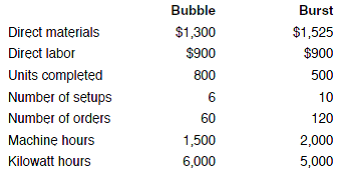REQUIREMENT Review the worksheet called ABC that follows these requirements. You have been asked to determine the cost of each product using an activity-based cost system. Note that the problem information is already entered into the Data Section of the ABC worksheet.
Free
Essay

The indicated worksheet should be reviewed. Cost of each product will be determined by activity-based cost system. The required worksheet is given below: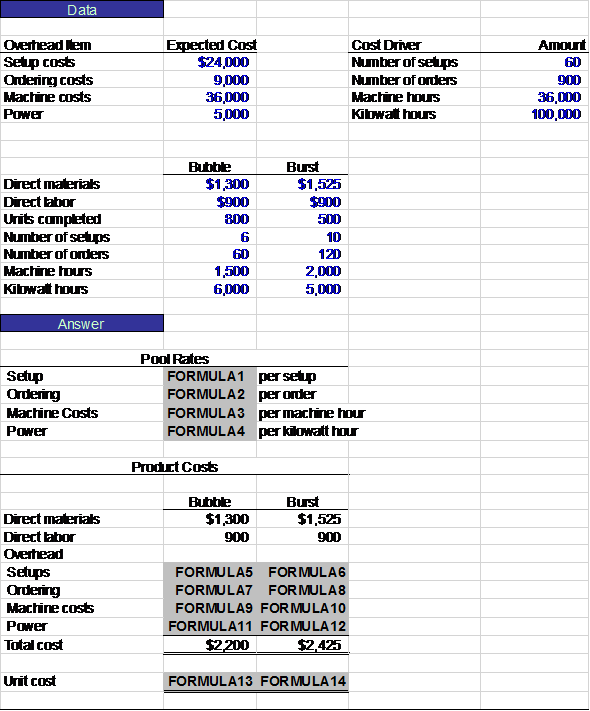TagsPROBLEM DATA Bumblebee Mobiles manufactures a line of cell phones. The management has identified the following overhead costs and related cost drivers for the coming year.The following were incurred in manufacturing two of their cell phones, Bubble and Burst, during the first quarter.REQUIREMENT Open the file ABC from the website for this book at cengagebrain.com. Enter the formulas in the appropriate cells on the worksheet. For example, FORMULA1 is =B8/E8. Enter your name in cell A1. Save your completed file as ABC2. Print the worksheet. Also print your formulas. Check figure: Cost per unit for Bubble (cell B43), $8.75. Free Essay Answer: Answer: The formulas are entered in the worksheet by using the cell reference. Rates are determined by dividing the number of the cost driver (setups, orders, etc.) by the total expected cost for that overhead item. Product costs are determined by multiplying the number of cost drivers for that product by the pool rate for that category. The required worksheet is given below: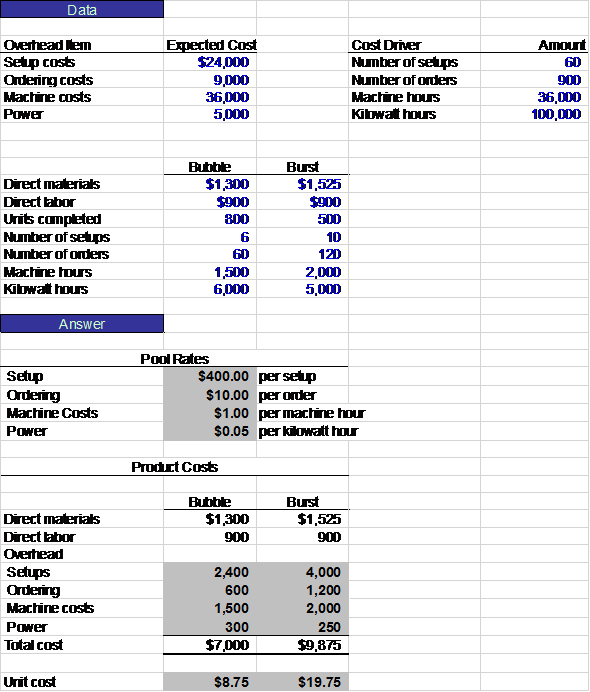TagsWHAT-IF ANALYSIS Bumblebee Mobiles is trying to reduce its unit costs. Management believes that the two easiest areas to save costs are setups and power. Determine the new cost information for each of the following independent situations. Remember to reset your data to the original information after answering each item. a. What are the new unit costs of each product if annual setup costs are reduced by 25% (Change cell B8.) Bubble:$________________ _ Burst: $________________ _ b. What is the new unit cost of each product if the number of setups can be reduced by 50% (Change cells B18 and C18.) Bubble:$________________ _ Burst: $________________ _ c. What is the new unit cost of each product if annual power costs are reduced by 70% (Change cell B11.) Bubble:$________________ _ Burst: $________________ _ Free Essay Answer: Answer: The management team for Company B is trying to reduce unit costs and believes that the easiest areas to save cost are setup and power. a. Annual setup costs are reduced by 25%. This will reduce the setup cost to$18,000. The calculation of unit cost is shown in the table given below: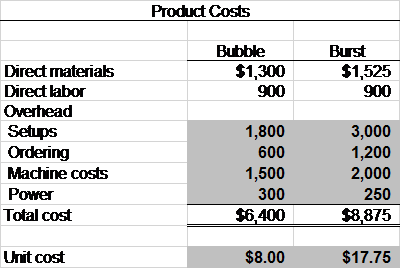b. The number of setups is reduced 50%. This will reduce the number of setups for Bubble to 3 and the number of setups for Burst to 5. The calculation of unit cost is shown in the table given below: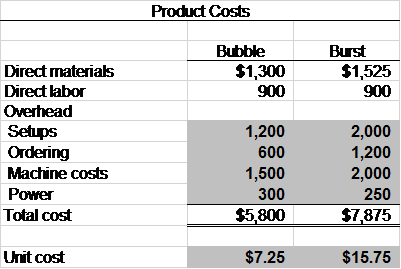c. Annual power costs are reduced by 70%. This will reduce the power cost to \$1,500. The calculation of unit cost is shown in the table given below: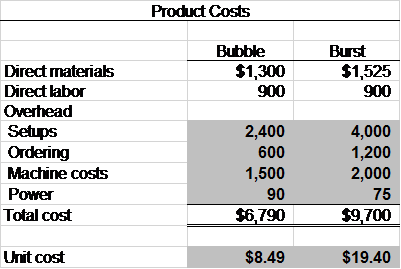TagsWHAT-IF ANALYSIS Which one of the cost saving ideas would create the lowest cost for each product Why
Essay